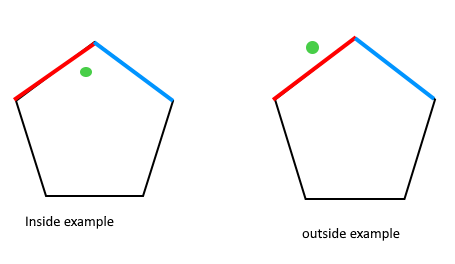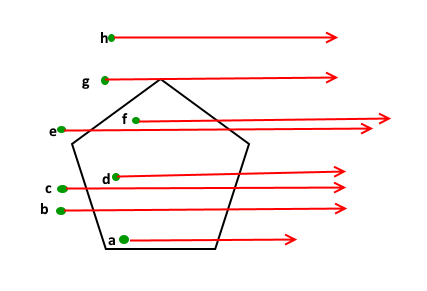Open in App
Not now

# How to check if a given point lies inside or outside a polygon?

• Difficulty Level : Hard
• Last Updated : 18 Jan, 2023

Given a polygon and a point ‘p‘, find if ‘p‘ lies inside the polygon or not. The points lying on the border are considered inside.

Examples:Approach: The idea to solve this problem is based on How to check if two given line segments intersect, and to be used as follows:

1. Draw a horizontal line to the right of each point and extend it to infinity
2. Count the number of times the line intersects with polygon edges.
3. A point is inside the polygon if either count of intersections is odd or point lies on an edge of polygon.  If none of the conditions is true, then point lies outside.How to handle point ‘g’ in the above figure?

Note that we should return true if the point lies on the line or the same as one of the vertices of the given polygon. To handle this, after checking if the line from ‘p’ to extreme intersects, we check whether ‘p’ is collinear with vertices of the current line of polygon. If it is collinear, then we check if the point ‘p’ lies on current side of polygon, if it lies, we return true, else false.

Following is the implementation of the above approach:

## C++

 `#include ``using` `namespace` `std;` `struct` `Point {``    ``int` `x, y;``};` `struct` `line {``    ``Point p1, p2;``};` `bool` `onLine(line l1, Point p)``{``    ``// Check whether p is on the line or not``    ``if` `(p.x <= max(l1.p1.x, l1.p2.x)``        ``&& p.x <= min(l1.p1.x, l1.p2.x)``        ``&& (p.y <= max(l1.p1.y, l1.p2.y)``            ``&& p.y <= min(l1.p1.y, l1.p2.y)))``        ``return` `true``;` `    ``return` `false``;``}` `int` `direction(Point a, Point b, Point c)``{``    ``int` `val = (b.y - a.y) * (c.x - b.x)``              ``- (b.x - a.x) * (c.y - b.y);` `    ``if` `(val == 0)` `        ``// Colinear``        ``return` `0;` `    ``else` `if` `(val < 0)` `        ``// Anti-clockwise direction``        ``return` `2;` `    ``// Clockwise direction``    ``return` `1;``}` `bool` `isIntersect(line l1, line l2)``{``    ``// Four direction for two lines and points of other line``    ``int` `dir1 = direction(l1.p1, l1.p2, l2.p1);``    ``int` `dir2 = direction(l1.p1, l1.p2, l2.p2);``    ``int` `dir3 = direction(l2.p1, l2.p2, l1.p1);``    ``int` `dir4 = direction(l2.p1, l2.p2, l1.p2);` `    ``// When intersecting``    ``if` `(dir1 != dir2 && dir3 != dir4)``        ``return` `true``;` `    ``// When p2 of line2 are on the line1``    ``if` `(dir1 == 0 && onLine(l1, l2.p1))``        ``return` `true``;` `    ``// When p1 of line2 are on the line1``    ``if` `(dir2 == 0 && onLine(l1, l2.p2))``        ``return` `true``;` `    ``// When p2 of line1 are on the line2``    ``if` `(dir3 == 0 && onLine(l2, l1.p1))``        ``return` `true``;` `    ``// When p1 of line1 are on the line2``    ``if` `(dir4 == 0 && onLine(l2, l1.p2))``        ``return` `true``;` `    ``return` `false``;``}` `bool` `checkInside(Point poly[], ``int` `n, Point p)``{` `    ``// When polygon has less than 3 edge, it is not polygon``    ``if` `(n < 3)``        ``return` `false``;` `    ``// Create a point at infinity, y is same as point p``    ``line exline = { p, { 9999, p.y } };``    ``int` `count = 0;``    ``int` `i = 0;``    ``do` `{` `        ``// Forming a line from two consecutive points of``        ``// poly``        ``line side = { poly[i], poly[(i + 1) % n] };``        ``if` `(isIntersect(side, exline)) {` `            ``// If side is intersects exline``            ``if` `(direction(side.p1, p, side.p2) == 0)``                ``return` `onLine(side, p);``            ``count++;``        ``}``        ``i = (i + 1) % n;``    ``} ``while` `(i != 0);` `    ``// When count is odd``    ``return` `count & 1;``}` `// Driver code``int` `main()``{``    ``Point polygon[]``        ``= { { 0, 0 }, { 10, 0 }, { 10, 10 }, { 0, 10 } };``    ``Point p = { 5, 3 };``    ``int` `n = 4;` `    ``// Function call``    ``if` `(checkInside(polygon, n, p))``        ``cout << ``"Point is inside."``;``    ``else``        ``cout << ``"Point is outside."``;``  ` `    ``return` `0;``}`

## Java

 `// Java code for the above approach``import` `java.util.*;` `public` `class` `Gfg {``  ``public` `static` `class` `Point {``    ``public` `int` `x, y;``    ``public` `Point(``int` `x, ``int` `y)``    ``{``      ``this``.x = x;``      ``this``.y = y;``    ``}``  ``}` `  ``public` `static` `class` `Line {``    ``public` `Point p1, p2;``    ``public` `Line(Point p1, Point p2)``    ``{``      ``this``.p1 = p1;``      ``this``.p2 = p2;``    ``}``  ``}` `  ``static` `int` `onLine(Line l1, Point p)``  ``{``    ``// Check whether p is on the line or not``    ``if` `(p.x <= Math.max(l1.p1.x, l1.p2.x)``        ``&& p.x <= Math.min(l1.p1.x, l1.p2.x)``        ``&& (p.y <= Math.max(l1.p1.y, l1.p2.y)``            ``&& p.y <= Math.min(l1.p1.y, l1.p2.y)))``      ``return` `1``;` `    ``return` `0``;``  ``}` `  ``static` `int` `direction(Point a, Point b, Point c)``  ``{``    ``int` `val = (b.y - a.y) * (c.x - b.x)``      ``- (b.x - a.x) * (c.y - b.y);` `    ``if` `(val == ``0``)` `      ``// Colinear``      ``return` `0``;` `    ``else` `if` `(val < ``0``)` `      ``// Anti-clockwise direction``      ``return` `2``;` `    ``// Clockwise direction``    ``return` `1``;``  ``}` `  ``static` `int` `isIntersect(Line l1, Line l2)``  ``{``    ``// Four direction for two lines and points of other``    ``// line``    ``int` `dir1 = direction(l1.p1, l1.p2, l2.p1);``    ``int` `dir2 = direction(l1.p1, l1.p2, l2.p2);``    ``int` `dir3 = direction(l2.p1, l2.p2, l1.p1);``    ``int` `dir4 = direction(l2.p1, l2.p2, l1.p2);` `    ``// When intersecting``    ``if` `(dir1 != dir2 && dir3 != dir4)``      ``return` `1``;` `    ``// When p2 of line2 are on the line1``    ``if` `(dir1 == ``0` `&& onLine(l1, l2.p1) == ``1``)``      ``return` `1``;` `    ``// When p1 of line2 are on the line1``    ``if` `(dir2 == ``0` `&& onLine(l1, l2.p2) == ``1``)``      ``return` `1``;` `    ``// When p2 of line1 are on the line2``    ``if` `(dir3 == ``0` `&& onLine(l2, l1.p1) == ``1``)``      ``return` `1``;` `    ``// When p1 of line1 are on the line2``    ``if` `(dir4 == ``0` `&& onLine(l2, l1.p2) == ``1``)``      ``return` `1``;` `    ``return` `0``;``  ``}` `  ``static` `int` `checkInside(Point[] poly, ``int` `n, Point p)``  ``{` `    ``// When polygon has less than 3 edge, it is not``    ``// polygon` `    ``if` `(n < ``3``)``      ``return` `0``;` `    ``// Create a point at infinity, y is same as point p``    ``Point pt = ``new` `Point(``9999``, p.y);``    ``Line exline = ``new` `Line(p, pt);``    ``int` `count = ``0``;``    ``int` `i = ``0``;``    ``do` `{` `      ``// Forming a line from two consecutive points of``      ``// poly``      ``Line side``        ``= ``new` `Line(poly[i], poly[(i + ``1``) % n]);``      ``if` `(isIntersect(side, exline) == ``1``) {` `        ``// If side is intersects exline``        ``if` `(direction(side.p1, p, side.p2) == ``0``)``          ``return` `onLine(side, p);``        ``count++;``      ``}``      ``i = (i + ``1``) % n;``    ``} ``while` `(i != ``0``);` `    ``// When count is odd``    ``return` `count & ``1``;``  ``}` `  ``// Driver code``  ``public` `static` `void` `main(String[] args)``  ``{``    ``Point[] polygon``      ``= { ``new` `Point(``1``, ``0``), ``new` `Point(``8``, ``3``),``         ``new` `Point(``8``, ``8``), ``new` `Point(``1``, ``5``) };``    ``Point p = ``new` `Point(``3``, ``5``);``    ``int` `n = polygon.length;``    ``if` `(checkInside(polygon, n, p) == ``1``)``      ``System.out.println(``"Point is Inside"``);``    ``else``      ``System.out.println(``"Point is Outside"``);``  ``}``}` `// This code is contributed by Potta Lokesh`

## Python3

 `class` `Point:``    ``def` `__init__(``self``, x, y):``        ``self``.x ``=` `x``        ``self``.y ``=` `y` `class` `line:``    ``def` `__init__(``self``, p1, p2):``        ``self``.p1 ``=` `p1``        ``self``.p2 ``=` `p2` `def` `onLine(l1, p):``    ``# Check whether p is on the line or not``    ``if` `(``        ``p.x <``=` `max``(l1.p1.x, l1.p2.x)``        ``and` `p.x <``=` `min``(l1.p1.x, l1.p2.x)``        ``and` `(p.y <``=` `max``(l1.p1.y, l1.p2.y) ``and` `p.y <``=` `min``(l1.p1.y, l1.p2.y))``    ``):``        ``return` `True``    ``return` `False` `def` `direction(a, b, c):``    ``val ``=` `(b.y ``-` `a.y) ``*` `(c.x ``-` `b.x) ``-` `(b.x ``-` `a.x) ``*` `(c.y ``-` `b.y)``    ``if` `val ``=``=` `0``:``        ``# Colinear``        ``return` `0``    ``elif` `val < ``0``:``        ``# Anti-clockwise direction``        ``return` `2``    ``# Clockwise direction``    ``return` `1` `def` `isIntersect(l1, l2):``    ``# Four direction for two lines and points of other line``    ``dir1 ``=` `direction(l1.p1, l1.p2, l2.p1)``    ``dir2 ``=` `direction(l1.p1, l1.p2, l2.p2)``    ``dir3 ``=` `direction(l2.p1, l2.p2, l1.p1)``    ``dir4 ``=` `direction(l2.p1, l2.p2, l1.p2)` `    ``# When intersecting``    ``if` `dir1 !``=` `dir2 ``and` `dir3 !``=` `dir4:``        ``return` `True` `    ``# When p2 of line2 are on the line1``    ``if` `dir1 ``=``=` `0` `and` `onLine(l1, l2.p1):``        ``return` `True` `    ``# When p1 of line2 are on the line1``    ``if` `dir2 ``=``=` `0` `and` `onLine(l1, l2.p2):``        ``return` `True` `    ``# When p2 of line1 are on the line2``    ``if` `dir3 ``=``=` `0` `and` `onLine(l2, l1.p1):``        ``return` `True` `    ``# When p1 of line1 are on the line2``    ``if` `dir4 ``=``=` `0` `and` `onLine(l2, l1.p2):``        ``return` `True` `    ``return` `False` `def` `checkInside(poly, n, p):``    ``# When polygon has less than 3 edge, it is not polygon``    ``if` `n < ``3``:``        ``return` `False` `    ``# Create a point at infinity, y is same as point p``    ``exline ``=` `line(p, Point(``9999``, p.y))``    ``count ``=` `0``    ``i ``=` `0``    ``while` `True``:``        ``# Forming a line from two consecutive points of poly``        ``side ``=` `line(poly[i], poly[(i ``+` `1``) ``%` `n])``        ``if` `isIntersect(side, exline):``            ``# If side is intersects ex``            ``if` `(direction(side.p1, p, side.p2) ``=``=` `0``):``                ``return` `onLine(side, p);``            ``count ``+``=` `1``        ` `        ``i ``=` `(i ``+` `1``) ``%` `n;``        ``if` `i ``=``=` `0``:``            ``break` `    ``# When count is odd``    ``return` `count & ``1``;`  `# Driver code``polygon ``=` `[  Point( ``0``, ``0` `), Point( ``10``, ``0` `), Point( ``10``, ``10` `), Point( ``0``, ``10` `) ];``p ``=` `Point( ``5``, ``3` `);``n ``=` `4``;` `# Function call``if` `(checkInside(polygon, n, p)):``    ``print``(``"Point is inside."``)``else``:``    ``print``(``"Point is outside."``)`

## C#

 `using` `System;``using` `System.Collections.Generic;` `public` `class` `Gfg``{``  ``public` `class` `Point {``    ``public` `int` `x, y;``    ``public` `Point(``int` `x, ``int` `y)``    ``{``      ``this``.x=x;``      ``this``.y=y;``    ``}``  ``}` `  ``public` `class` `line {``    ``public` `Point p1, p2;``    ``public` `line(Point p1, Point p2)``    ``{``      ``this``.p1=p1;``      ``this``.p2=p2;``    ``}``  ``}` `  ``static` `int` `onLine(line l1, Point p)``  ``{``    ``// Check whether p is on the line or not``    ``if` `(p.x <= Math.Max(l1.p1.x, l1.p2.x)``        ``&& p.x <= Math.Min(l1.p1.x, l1.p2.x)``        ``&& (p.y <= Math.Max(l1.p1.y, l1.p2.y)``            ``&& p.y <= Math.Min(l1.p1.y, l1.p2.y)))``      ``return` `1;` `    ``return` `0;``  ``}` `  ``static` `int` `direction(Point a, Point b, Point c)``  ``{``    ``int` `val = (b.y - a.y) * (c.x - b.x)``      ``- (b.x - a.x) * (c.y - b.y);` `    ``if` `(val == 0)` `      ``// Colinear``      ``return` `0;` `    ``else` `if` `(val < 0)` `      ``// Anti-clockwise direction``      ``return` `2;` `    ``// Clockwise direction``    ``return` `1;``  ``}` `  ``static` `int` `isIntersect(line l1, line l2)``  ``{``    ``// Four direction for two lines and points of other line``    ``int` `dir1 = direction(l1.p1, l1.p2, l2.p1);``    ``int` `dir2 = direction(l1.p1, l1.p2, l2.p2);``    ``int` `dir3 = direction(l2.p1, l2.p2, l1.p1);``    ``int` `dir4 = direction(l2.p1, l2.p2, l1.p2);` `    ``// When intersecting``    ``if` `(dir1 != dir2 && dir3 != dir4)``      ``return` `1;` `    ``// When p2 of line2 are on the line1``    ``if` `(dir1 == 0 && onLine(l1, l2.p1)==1)``      ``return` `1;` `    ``// When p1 of line2 are on the line1``    ``if` `(dir2 == 0 && onLine(l1, l2.p2)==1)``      ``return` `1;` `    ``// When p2 of line1 are on the line2``    ``if` `(dir3 == 0 && onLine(l2, l1.p1)==1)``      ``return` `1;` `    ``// When p1 of line1 are on the line2``    ``if` `(dir4 == 0 && onLine(l2, l1.p2)==1)``      ``return` `1;` `    ``return` `0;``  ``}` `  ``static` `int` `checkInside(Point[] poly, ``int` `n, Point p)``  ``{` `    ``// When polygon has less than 3 edge, it is not polygon``    ``if` `(n < 3)``      ``return` `0;` `    ``// Create a point at infinity, y is same as point p``    ``Point pt=``new` `Point(9999, p.y);``    ``line exline = ``new` `line(p,pt);``    ``int` `count = 0;``    ``int` `i = 0;``    ``do` `{` `      ``// Forming a line from two consecutive points of``      ``// poly``      ``line side = ``new` `line( poly[i], poly[(i + 1) % n] );``      ``if` `(isIntersect(side, exline)==1) {` `        ``// If side is intersects exline``        ``if` `(direction(side.p1, p, side.p2) == 0)``          ``return` `onLine(side, p);``        ``count++;``      ``}``      ``i = (i + 1) % n;``    ``} ``while` `(i != 0);` `    ``// When count is odd``    ``return` `count & 1;``  ``}` `  ``// Driver code``  ``public` `static` `void` `Main(``string``[] args)``  ``{``    ``Point[] polygon``      ``= { ``new` `Point(0, 0 ), ``new` `Point(10, 0 ), ``new` `Point(10, 10 ), ``new` `Point(0, 10 ) };``    ``Point p = ``new` `Point(5, 3 );``    ``int` `n = 4;` `    ``// Function call``    ``if` `(checkInside(polygon, n, p)==1)``      ``Console.WriteLine(``"Point is inside."``);``    ``else``      ``Console.WriteLine(``"Point is outside."``);``  ``}` `}` `// This code is contributed by ratiagarawal.`

## Javascript

 `class Point {``    ``//int x, y;``    ``constructor(x,y)``    ``{``        ``this``.x=x;``        ``this``.y=y;``    ``}``}` `class line {``    ``//Point p1, p2;``    ``constructor(p1,p2)``    ``{``        ``this``.p1=p1;``        ``this``.p2=p2;``    ``}` `};` `function` `onLine(l1, p)``{``    ``// Check whether p is on the line or not``    ``if` `(p.x <= Math.max(l1.p1.x, l1.p2.x)``        ``&& p.x <= Math.min(l1.p1.x, l1.p2.x)``        ``&& (p.y <= Math.max(l1.p1.y, l1.p2.y)``            ``&& p.y <= Math.min(l1.p1.y, l1.p2.y)))``        ``return` `true``;` `    ``return` `false``;``}` `function` `direction(a, b, c)``{``    ``let val = (b.y - a.y) * (c.x - b.x)``              ``- (b.x - a.x) * (c.y - b.y);` `    ``if` `(val == 0)` `        ``// Colinear``        ``return` `0;` `    ``else` `if` `(val < 0)` `        ``// Anti-clockwise direction``        ``return` `2;` `    ``// Clockwise direction``    ``return` `1;``}` `function` `isIntersect(l1, l2)``{``    ``// Four direction for two lines and points of other line``    ``let dir1 = direction(l1.p1, l1.p2, l2.p1);``    ``let dir2 = direction(l1.p1, l1.p2, l2.p2);``    ``let dir3 = direction(l2.p1, l2.p2, l1.p1);``    ``let dir4 = direction(l2.p1, l2.p2, l1.p2);` `    ``// When intersecting``    ``if` `(dir1 != dir2 && dir3 != dir4)``        ``return` `true``;` `    ``// When p2 of line2 are on the line1``    ``if` `(dir1 == 0 && onLine(l1, l2.p1))``        ``return` `true``;` `    ``// When p1 of line2 are on the line1``    ``if` `(dir2 == 0 && onLine(l1, l2.p2))``        ``return` `true``;` `    ``// When p2 of line1 are on the line2``    ``if` `(dir3 == 0 && onLine(l2, l1.p1))``        ``return` `true``;` `    ``// When p1 of line1 are on the line2``    ``if` `(dir4 == 0 && onLine(l2, l1.p2))``        ``return` `true``;` `    ``return` `false``;``}` `function` `checkInside(poly, n, p)``{` `    ``// When polygon has less than 3 edge, it is not polygon``    ``if` `(n < 3)``        ``return` `false``;` `    ``// Create a point at infinity, y is same as point p``    ``let tmp=``new` `Point(9999, p.y);``    ``let exline = ``new` `line( p, tmp );``    ``let count = 0;``    ``let i = 0;``    ``do` `{` `        ``// Forming a line from two consecutive points of``        ``// poly``        ``let side = ``new` `line( poly[i], poly[(i + 1) % n] );``        ``if` `(isIntersect(side, exline)) {` `            ``// If side is intersects exline``            ``if` `(direction(side.p1, p, side.p2) == 0)``                ``return` `onLine(side, p);``            ``count++;``        ``}``        ``i = (i + 1) % n;``    ``} ``while` `(i != 0);` `    ``// When count is odd``    ``return` `count & 1;``}` `// Driver code``    ``let polygon= [ ``new` `Point(0, 0 ), ``new` `Point(10, 0 ), ``new` `Point(10, 10 ),  ``new` `Point(0, 10) ];``    ``let p = ``new` `Point( 5, 3 );``    ``let n = 4;` `    ``// Function call``    ``if` `(checkInside(polygon, n, p))``        ``console.log(``"Point is inside."``);``    ``else``        ``console.log(``"Point is outside."``);`` ` ` ``// This code is contributed by poojaagarwal2.`

Output

`Point is inside.`

Time Complexity: O(n) where n is the number of vertices in the given polygon.
Auxiliary Space: O(1), since no extra space has been taken.